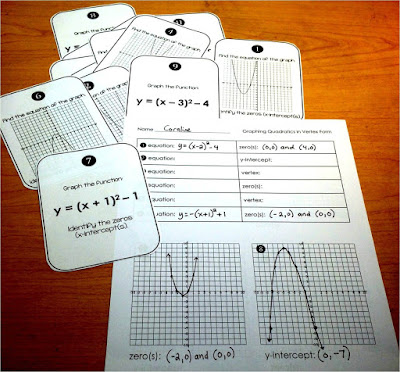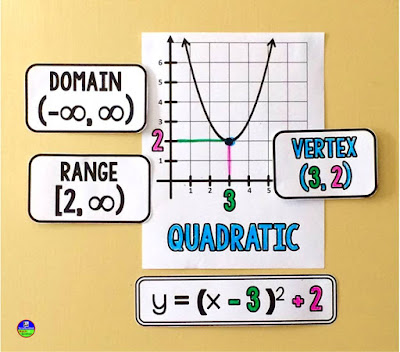## Pages

### Vertex schmertex (vertex form quadratics)

We started graphing and writing equations for vertex form quadratics in my Algebra 2 classes this week. Well, half my students did - the other half were re-taking the state exit exam. Don't get me started...

Because half of my students missed most of the introduction to the unit, I needed a basic activity that would allow some students to work independently while I worked more one-on-one with the others who had been out.Vertex form quadratics task cards

So I made 12 task cards that ask students to graph and write equations of vertex form quadratics. Every task card has an additional question on it like "find the zeros" or "find the y-intercept" to keep skills up.Graphing quadratics in vertex form cheat sheet

I had given this reference sheet to my students to help them remember the steps for graphing vertex form quadratics. They keep the sheet in their notebooks (it's a 1/2 sheet) to use during class.

And here is a video that goes along with the sheet.Part of an Algebra 2 word wall

On our classroom walls are lots of word wall references. We use our vertex form example posters (part of our Algebra 2 word wall) probably the most of any other reference. Above are the parts of our reference for radicals, which come up later.

You can find the graphing quadratics cheat sheet here

Subscribe to blog for more math freebies

I teach:

1.1.